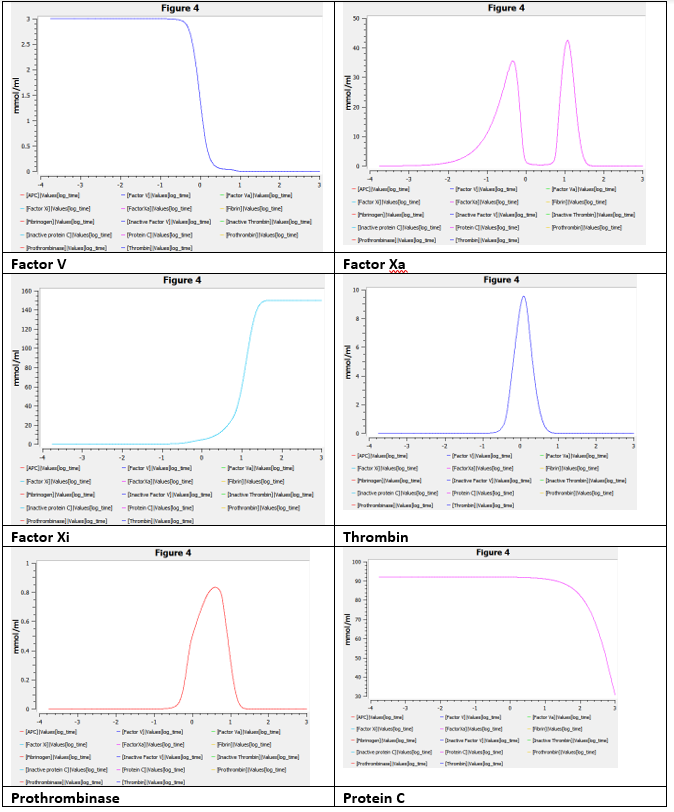## Dunster2016 - Nondimensional Coagulation ModelModel Identifier
BIOMD0000000925
Short description
We undertake a mathematical investigation of a model for the generation of thrombin, an enzyme central to haemostatic blood coagulation, as well as to thrombotic disorders, that is the end product of a complicated protein cascade with multiple feedbacks that ensures its production in the right place at the right time. In a laboratory setting, its central role is reflected in thrombin evolution over time being used as a measure of the ability of a patient's blood to clot. Here, we present a model for the generation of thrombin (based on earlier work) and analyse it using the method of matched asymptotic expansions to derive a sequence of simplified models that characterize the roles of distinct interactions over various timescales. In particular, we are able through the asymptotic analysis to provide simplified models that are an excellent substitute for the full model (capturing the explosive growth and decay of thrombin) and approximations for the key experimental measurements used to describe thrombin's characteristic evolution over time. The asymptotic results are validated against numerical simulations.
Format
SBML (L2V4)
Related Publication
• Mathematical modelling of thrombin generation: asymptotic analysis and pathway characterization• J. L. Dunster, J. R. King
• IMA Journal of Applied Mathematics , 2/ 2017 , Volume 82 , Issue 1 , pages: 60-96 , DOI: 10.1093/imamat/hxw007
• Department of Mathematics and Statistics, Institute for Cardiovascular and Metabolic Research, School of Biological Sciences, University of Reading, Reading, UK.
• We undertake a mathematical investigation of a model for the generation of thrombin, an enzyme central to haemostatic blood coagulation, as well as to thrombotic disorders, that is the end product of a complicated protein cascade with multiple feedbacks that ensures its production in the right place at the right time. In a laboratory setting, its central role is reflected in thrombin evolution over time being used as a measure of the ability of a patient's blood to clot. Here, we present a model for the generation of thrombin (based on earlier work) and analyse it using the method of matched asymptotic expansions to derive a sequence of simplified models that characterize the roles of distinct interactions over various timescales. In particular, we are able through the asymptotic analysis to provide simplified models that are an excellent substitute for the full model (capturing the explosive growth and decay of thrombin) and approximations for the key experimental measurements used to describe thrombin's characteristic evolution over time. The asymptotic results are validated against numerical simulations.
Contributors
Submitter of the first revision: Matthew Roberts
Submitter of this revision: Ahmad Zyoud

is (3 statements)
BioModels Database MODEL1808140001
BioModels Database MODEL1808140001
BioModels Database BIOMD0000000925

hasTaxon (1 statement)
Taxonomy Homo sapiens

isVersionOf (1 statement)
Gene Ontology blood coagulation

hasProperty (1 statement)
Mathematical Modelling Ontology Ordinary differential equation model

occursIn (1 statement)
Brenda Tissue Ontology blood

isDescribedBy (1 statement)

Curation status
Curated

Modelling approach(es)

Tags

#### Connected external resources

SBGN view in Newt Editor

Name Description Size Actions

### Model files

Dunster2016_Nondimensional_Model_Curated.xml SBML L2V4 representation of Dunster2016 - Nondimensional Coagulation Model_Curated-Figure 4 178.02 KB Preview | Download

Dunster2016_Dimensional_Model.cps Model COPASI file for dimensional model. 97.54 KB Preview | Download
Dunster2016_Nondimensional_Model (1).xml SBML L2V4 representation of Dunster2016 - Nondimensional Coagulation Model_Orignal 32.31 KB Preview | Download
Dunster2016_Nondimensional_Model.cps Curated (nondimensional) model COPASI file. Figure 4 simulation results reproduced. 85.55 KB Preview | Download
Dunster2016_Nondimensional_Model1.sedml sed-ml L1V2 representation of Dunster2016 - Nondimensional Coagulation Model_Curated-Figure 4 14.96 KB Preview | Download
Dunster2016_Nondimensional_Model_Curated.cps COPASI version 4.27 (Build 217) representation of Dunster2016 - Nondimensional Coagulation Model_Curated-Figure 4 295.14 KB Preview | Download
• Model originally submitted by : Matthew Roberts
• Submitted: Aug 14, 2018 2:32:35 PM
##### Revisions

(*) You might be seeing discontinuous revisions as only public revisions are displayed here. Any private revisionsof this model will only be shown to the submitter and their collaborators.

Legends
: Variable used inside SBML models

Species
Species Initial Concentration/Amount
Xa L 0.0 mmol
Xa 0.0 mmol
Va Xa L 0.0 mmol
Va 0.0 mmol
PC 92.0 mmol
IIa ATIII 0.0 mmol
II 17.0 mmol
Fibrin 0.0 mmol
Xa ATIII 0.0 mmol
IIa 0.0 mmol
Reactions
Reactions Rate Parameters
Xa_L = 0.5*((k_tilde_x+l_tilde_x+Xa)-((k_tilde_x+l_tilde_x+Xa)^2-4*l_tilde_x*Xa)^(0.5)) [] k_tilde_x = 385.0; l_tilde_x = 7.69
Xa = ((k_tilde_1a*gamma_tilde_1a*exp((-gamma_tilde_1a)*time)+k_tilde_3c*k_tilde_3a*APC*Va_Xa/(Va_Xa+1))-k_tilde_1b*Xa)-k_tilde_3a*Xa*Va ((k_tilde_1a*gamma_tilde_1a*exp((-gamma_tilde_1a)*time)+k_tilde_3c*k_tilde_3a*APC*Va_Xa/(Va_Xa+1))-k_tilde_1b*Xa)-k_tilde_3a*Xa*Va gamma_tilde_1a = 0.77; k_tilde_3a = 150.0; k_tilde_1a = 150.0; k_tilde_3c = 1.0; k_tilde_1b = 0.19
Va_Xa_L = 0.5*((k_tilde_b+l_tilde_b+Va_Xa)-((k_tilde_b+l_tilde_b+Va_Xa)^2-4*l_tilde_b*Va_Xa)^(0.5)) [] k_tilde_b = 5.0E-4; l_tilde_b = 0.05
Va = ((IIa*V/(V+k_tilde_2am*(1+Fibrinogen))+k_tilde_2b*Xa*V/(V+1+II)+k_tilde_3b/q_tilde_3a*Va_Xa)-APC*Va/(Va+1))-Xa*Va ((IIa*V/(V+k_tilde_2am*(1+Fibrinogen))+k_tilde_2b*Xa*V/(V+1+II)+k_tilde_3b/q_tilde_3a*Va_Xa)-APC*Va/(Va+1))-Xa*Va q_tilde_3a = 1.0; k_tilde_2am = 7.2; k_tilde_2b = 0.013; k_tilde_3b = 0.038
PC = (-k_tilde_5a)*PC (-k_tilde_5a)*PC k_tilde_5a = 0.0011
IIa_ATIII = IIa IIa []
II = (-q_tilde_4a)*Xa_L*II/(V+1+II)-k_tilde_4b*Va_Xa_L*II/(II+k_tilde_4bm) (-q_tilde_4a)*Xa_L*II/(V+1+II)-k_tilde_4b*Va_Xa_L*II/(II+k_tilde_4bm) k_tilde_4b = 530.0; q_tilde_4a = 0.004; k_tilde_4bm = 3.6
Fibrin = k_tilde_6*Fibrinogen k_tilde_6*Fibrinogen k_tilde_6 = 1500.0
Xa_ATIII = k_tilde_1b*Xa+k_tilde_3a*k_tilde_3b/q_tilde_3a*Va_Xa k_tilde_1b*Xa+k_tilde_3a*k_tilde_3b/q_tilde_3a*Va_Xa k_tilde_3a = 150.0; q_tilde_3a = 1.0; k_tilde_3b = 0.038; k_tilde_1b = 0.19
IIa = (k_tilde_4a*Xa_L*II/(V+1+II)+k_tilde_4a*k_tilde_4b*Va_Xa_L*II/(q_tilde_4a*(II+k_tilde_4bm)))-IIa (k_tilde_4a*Xa_L*II/(V+1+II)+k_tilde_4a*k_tilde_4b*Va_Xa_L*II/(q_tilde_4a*(II+k_tilde_4bm)))-IIa k_tilde_4b = 530.0; k_tilde_4a = 0.12; q_tilde_4a = 0.004; k_tilde_4bm = 3.6Curator's comment:
(added: 26 Mar 2020, 14:50:26, updated: 26 Mar 2020, 14:50:26)
All the figures within the Figure 4 has been successfully reproduced except of a slight shift in the Y-axis